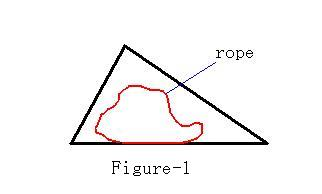Online JudgeProblem SetAuthorsOnline ContestsUser
Web Board
F.A.Qs
Statistical Charts
Problems
Submit Problem
Online Status
Prob.ID:
Register
Authors ranklist
Current Contest
Past Contests
Scheduled Contests
Award Contest
Register
Language:
Area in Triangle
 Time Limit: 1000MS Memory Limit: 30000K Total Submissions: 1914 Accepted: 894

Description

Given a triangle field and a rope of a certain length (Figure-1), you are required to use the rope to enclose a region within the field and make the region as large as possible.Input

The input has several sets of test data. Each set is one line containing four numbers separated by a space. The first three indicate the lengths of the edges of the triangle field, and the fourth is the length of the rope. Each of the four numbers have exactly four digits after the decimal point. The line containing four zeros ends the input and should not be processed. You can assume each of the edges are not longer than 100.0000 and the length of the rope is not longer than the perimeter of the field.

Output

Output one line for each case in the following format:

Case i: X

Where i is the case number, and X is the largest area which is rounded to two digits after the decimal point.

Sample Input

```12.0000 23.0000 17.0000 40.0000
84.0000 35.0000 91.0000 210.0000
100.0000 100.0000 100.0000 181.3800
0 0 0 0
```

Sample Output

```Case 1: 89.35
Case 2: 1470.00
Case 3: 2618.00
```

Source

[Submit]   [Go Back]   [Status]   [Discuss]Home PageGo BackTo top